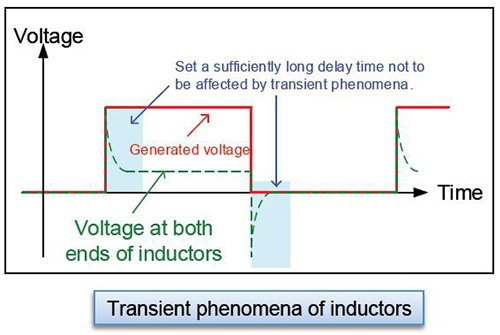# Inductors (Coils)

## What are inductors or coils?

Coils may be coreless (having an air core or a core made of a non-magnetic metal), or they may have a core made of a magnetic metal (i.e., a metal with high magnetic permeability) such as ferrite. Inductors with cores exhibit current dependence.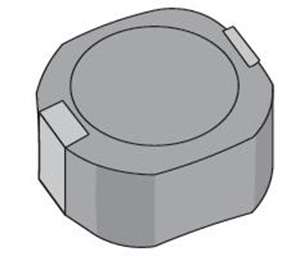## Setting example of measurement conditions

The phenomenon of LC resonance with the coil’s (inductor’s) inductance and parasitic capacitance is known as self-resonance. The frequency at which self-resonance occurs is known as the self-resonant frequency. When evaluating coils, be sure to measure L and Q at a frequency that is sufficiently lower than the self-resonant frequency.
A coil’s inductance, which increases with frequency, can be calculated using the following equation: Z=j2πfL. To measure inductance efficiently while varying the frequency, set the measurement range to AUTO. To measure with a higher degree of precision, set the frequency to produce an impedance that can be measured with a high-accuracy range.

## Setting the measurement frequency

The phenomenon of LC resonance with the coil’s (inductor’s) inductance and parasitic capacitance is known as self-resonance. The frequency at which self-resonance occurs is known as the self-resonant frequency. When evaluating coils, be sure to measure L and Q at a frequency that is sufficiently lower than the self-resonant frequency.

A coil’s inductance, which increases with frequency, can be calculated using the following equation: Z=j2πfL. To measure inductance efficiently while varying the frequency, set the measurement range to AUTO. To measure with a higher degree of precision, set the frequency to produce an impedance that can be measured with a high-accuracy range.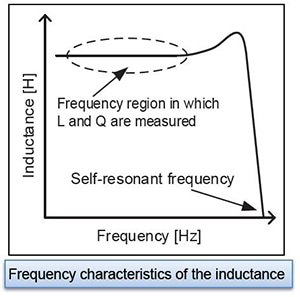## Setting the measurement signal level

The measurement current can be calculated from the open-terminal voltage, the instrument’s output impedance, and the measurement target’s impedance. Set the measurement voltage so that the rated current is not exceeded.

When measuring a coil that exhibits current dependence (i.e., a coil with a magnetic core), set the instrument to a signal level such that the magnetic core is not saturated. When measuring a coil that does not exhibit current dependence, it is recommended to set the instrument to the signal level with the best accuracy. With the IM35xx series, the best accuracy is achieved with the V mode’s 1 V setting. With the IM758x series, the measurement signal level is defined for the power when using the DUT port’s 50 Ω termination, and the setting with the best accuracy is +1 dBm.

When measuring a coil with a core or a coil with a low rated current, the IM35xx series’ CC (constant current) mode is convenient. The measurement current is controlled in software so that it remains constant.## Instruments for Mass Production Applications

 Model Measurement frequency Features IM3533 DC,1mHz to 200kHz Temperature correction function of Rdc IM3536 DC,4Hz to 8MHz Standard model,high-speed,highly stable, cost-effective analyzer IM7581 100kHz to 300MHz High-speed measurement of coils for high frequency

## Instruments for Research & Development Applications

 Model Measurement frequency Features IM3570 DC,4Hz to 5MHz Frequency sweep with analyzer mode

## Selecting Parameter, Ls or Lp

The table on the right shows impedance according to frequency (when D is sufficiently small)

Generally speaking, series equivalent circuit mode is used when measuring low-impedance elements (approximately 100Ω or less), and parallel equivalent circuit mode is used when measuring high-impedance elements (approximately 10 kΩ or greater). When the appropriate equivalent circuit mode is unclear, for example when measuring a sample with an impedance from approximately 100Ω to 10 kΩ, check with the component’s manufacturer.

An inductor will behave as though the winding’s copper loss Rs and the core loss Rp have been connected to an ideal inductor L. An ideal coil’s inductance can be calculated as follows: XL＝j2πfL. Although no general formulation is possible since it varies with the magnitude of Rs and Rp, low-inductance coils are characterized by a small XL, allowing the impedance when Rp and L are placed in parallel to be treated as roughly equivalent to XL. Rs can be ignored since Ls is small, so the series equivalent circuit is used. By contrast, when the impedance is high, Rp cannot be ignored but Rs can, so the setup can be treated as a parallel equivalent circuit.

•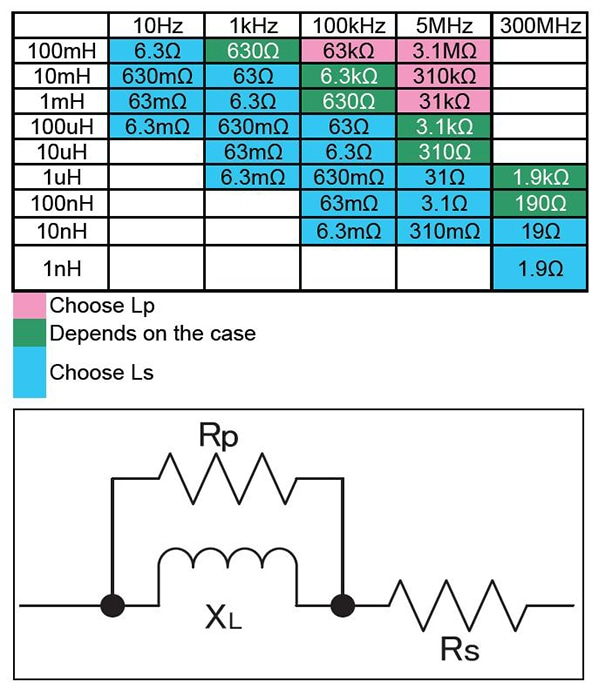### Diagram: Equivalent circuit of inductors

*Low-inductance coils: Rp can be ignored since impedance is low. Select series equivalent circuit modes.
*High-inductance coils: Rs can be ignored since impedance is high.Select series equivalent circuit modes.

## Current flowing to the coil

The current flowing to the coil can be calculated based on the open-terminal voltage, the instrument’s output impedance, and the measurement target’s impedance.

*1 The output impedance varies depending on the model and on whether low-impedance high-precision mode has been enabled. Please refer to the product specifications in the instruction manual.

•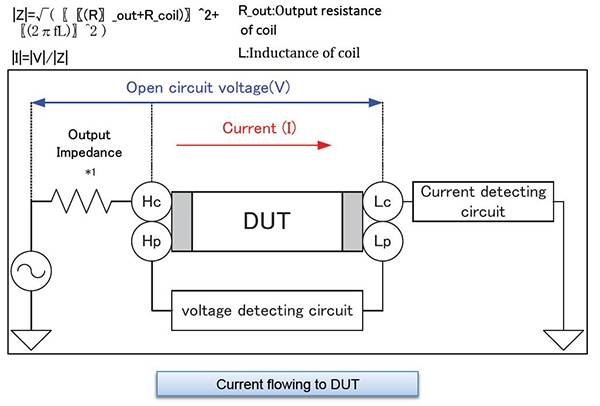## Measuring Rdc

In coil evaluation, L, Q, and Rdc are measured. Instruments such as the IM3533 and IM3536 can measure L, Q, and Rdc without the need to use any other devices. After measuring L and Q with an AC signal, measure Rdc with a DC signal.
*Rs and Rp are not equal to Rdc. Rs and Rp are resistance values that are measured with an AC signal. They include components such as coil loss and winding resistance, which increases due to conductor skin effects and proximity effects.
When the winding material has a large temperature coefficient, Rdc will vary with temperature. The
IM3533 has temperature correction functionality for Rdc.

•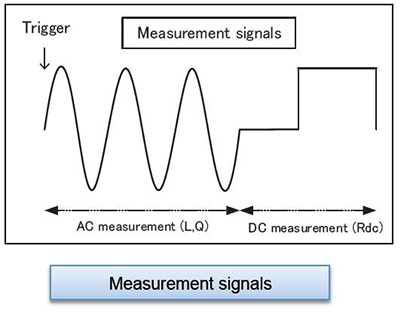## DC superposition characteristics

Coil characteristics include DC superposition characteristics, which indicate the extent to which inductance decreases relative to DC current, an important evaluation item for coils that will be used in circuits such as power supply circuits that handle large currents.

The DC bias voltage application function built into Hioki LCR meters is designed for use in measuring capacitors, and it cannot be used to apply a DC current. To superpose a DC signal, either use the DC Bias Current Unit 9269 (or 9269-10) and an external power supply, or create your own circuit for the purpose.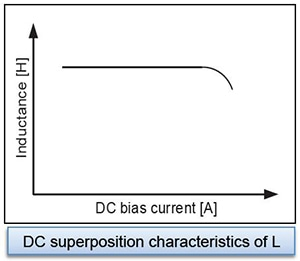## Setting the delay time

To reduce measurement error during Rdc measurement, Hioki LCR meters cycle the generated voltage on and off to cancel the internal offset (DC adjustment function).

When the voltage being applied to the inductor changes, the output resistance and inductor’s equivalent series resistance and inductance cause transient phenomena. Set a sufficiently long delay time during Rdc measurement to ensure that the measurement results are not affected by these phenomena. The name given to the delay time setting varies by model, as does measurement timing. For more information, please see the instruction manual for the model you intend to use.

•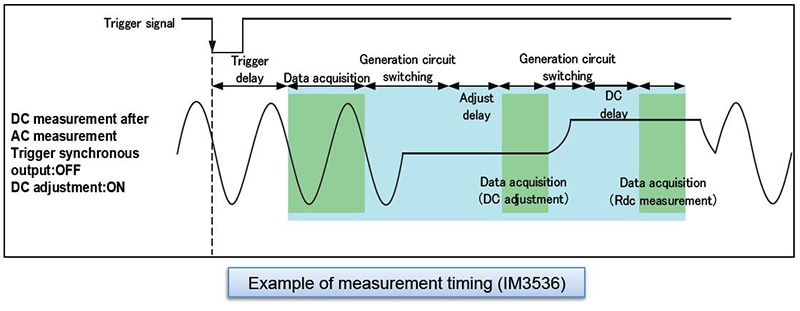## Setting the delay time - reducing variability

If you are unsure of the appropriate delay time, first set as long a delay time as possible. Then gradually shorten the delay time while verifying that measured values do not exhibit any variability.

•